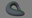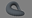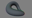I have had a geometry shader for visualizing normals for quite some time now, and I thought about sharing it because it's quite a handy little debugging tool!

The output of the geometry shader, is lines going from the vertex and in the direction of the normal of that vertex. This allows you to see if the normals are indeed correct and if they're normalized.

The geometry shader have multiple features. So I've added multiple version of it to this post. So you can use the version that fits your needs and save performance on the features you don't need!

###### § Features
• Change the length of the outputted lines.
• Uniform coloring or colored using the normals.
• Max render distance.
• Rotated models and correct normal coloring.## § Rendering

You can use any primitive type you want, as long as you draw it using `GL_POINTS` when visualizing the normals:

```glDrawArrays(GL_POINTS, ...);
// or
glDrawElements(GL_POINTS, ...);
```

Essentially when using the shader, do something like this:

```// Draw the mesh as you would normally
glUseProgram(program);
glDrawArrays(primitiveType, ...);

// Visualize the normals
glUseProgram(programVisualizeNormals);
glDrawArrays(GL_POINTS, ...);
```

## § Vertex & Fragment Shaders

No matter which version of the geometry shader you chose. The vertex shader and the fragment/pixel shader stays the same!

Change the attributes, so it matches your own layout.

### § Vertex Shader

```#version 330 core

layout(location = 0) in vec3 position;
layout(location = 1) in vec3 normal;

out vec3 vertex_normal;

void main()
{
vertex_normal = normal;

gl_Position = vec4(position, 1.0);
}
```

### § Fragment Shader

```#version 330 core

layout(location = 0) out vec4 frag_color;

in vec3 vertex_color;

void main()
{
frag_color = vec4(vertex_color, 1.0);
}
```

## § Geometry Shader (Uniform Colored)

This is the most basic version. It outputs lines in the color of `uniform vec3 color;`, and it also allows you to change the length of the outputted lines, by changing `uniform length;`.

```#version 330 core

layout(points) in;
layout(line_strip, max_vertices = 2) out;

uniform mat4 mvp; // Model View Projection Matrix

uniform float length = 1.0;
uniform vec3 color = vec3(1.0, 1.0, 1.0);

in vec3 vertex_normal[];

out vec3 vertex_color;

void main()
{
vec3 normal = vertex_normal;

vertex_color = color;

vec4 v0 = gl_in.gl_Position;
gl_Position = mvp * v0;
EmitVertex();

vec4 v1 = v0 + vec4(normal * length, 0.0);
gl_Position = mvp * v1;
EmitVertex();

EndPrimitive();
}
```## § Geometry Shader (Normal Colored)

This version colors all the outputted lines in the color of `abs(normal)`. Thereby making it easier to see what the normal values actually are.

If you intend to use this version, then scroll down and read the Rotated Models part as well.

```#version 330 core

layout(points) in;
layout(line_strip, max_vertices = 2) out;

uniform mat4 mvp; // Model View Projection Matrix

uniform float length = 1.0;

in vec3 vertex_normal[];

out vec3 vertex_color;

void main()
{
vec3 normal = vertex_normal;

vertex_color = abs(normal);

vec4 v0 = gl_in.gl_Position;
gl_Position = mvp * v0;
EmitVertex();

vec4 v1 = v0 + vec4(normal * length, 0.0);
gl_Position = mvp * v1;
EmitVertex();

EndPrimitive();
}
```## § Geometry Shader (Max Render Distance)

This version is much better if you're rendering a lot of vertices. As this version allows you to set/change a `max_render_distance` uniform, which limits the amount of outputted lines.

It renders normals from the camera and out to `max_render_distance`, thereby saving processing for not having to generate lines for vertices far away from the camera.

If you intend to use this version, then scroll down and read the Rotated Models part as well.

```#version 330 core

layout(points) in;
layout(line_strip, max_vertices = 2) out;

uniform mat4 mvp; // Model View Projection Matrix

uniform float length = 1.0;
uniform float max_render_distance = 100.0;

in vec3 vertex_normal[];

out vec3 vertex_color;

void main()
{
vec4 v0 = gl_in.gl_Position;
gl_Position = mvp * v0;

if (length(gl_Position) < max_render_distance)
{
vec3 normal = vertex_normal;

vertex_color = abs(normal);

EmitVertex();

vec4 v1 = v0 + vec4(normal * length, 0.0);
gl_Position = mvp * v1;
EmitVertex();

EndPrimitive();
}
}
```## § Rotated Models

This is only important if you're rendering normals on a rotated model and if the outputted lines are colored using the normals. (Like the following deformed rotating torus).

If the model is rotated, then we need to rotate the normals as well. If we don't do that, then the line colors aren't going to be correct! Notice how in the video, the lines pointing left and right always stays reddish. That's because they're rotated correctly!

The only thing you need is to add another uniform and change a single line of code in the geometry shader!

The uniform we need to add, is for the normal matrix:

```uniform mat4 nm; // normal matrix = transpose(inverse(model))
```

Then we need to multiply the normal to the normal matrix, so change this:

```vertex_color = abs(normal);
```

To this:

```vertex_color = abs(vec4(nm * vec4(vertex_normal, 0.0)).xyz);
```